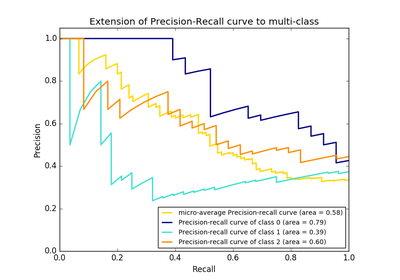# `sklearn.metrics`.average_precision_score¶

`sklearn.metrics.``average_precision_score`(y_true, y_score, average='macro', sample_weight=None)[source]

Compute average precision (AP) from prediction scores

This score corresponds to the area under the precision-recall curve.

Note: this implementation is restricted to the binary classification task or multilabel classification task.

Read more in the User Guide.

Parameters: y_true : array, shape = [n_samples] or [n_samples, n_classes] True binary labels in binary label indicators. y_score : array, shape = [n_samples] or [n_samples, n_classes] Target scores, can either be probability estimates of the positive class, confidence values, or non-thresholded measure of decisions (as returned by “decision_function” on some classifiers). average : string, [None, ‘micro’, ‘macro’ (default), ‘samples’, ‘weighted’] If `None`, the scores for each class are returned. Otherwise, this determines the type of averaging performed on the data: `'micro'`: Calculate metrics globally by considering each element of the label indicator matrix as a label. `'macro'`: Calculate metrics for each label, and find their unweighted mean. This does not take label imbalance into account. `'weighted'`: Calculate metrics for each label, and find their average, weighted by support (the number of true instances for each label). `'samples'`: Calculate metrics for each instance, and find their average. sample_weight : array-like of shape = [n_samples], optional Sample weights. average_precision : float

`roc_auc_score`
Area under the ROC curve
`precision_recall_curve`
Compute precision-recall pairs for different probability thresholds

References

Examples

```>>> import numpy as np
>>> from sklearn.metrics import average_precision_score
>>> y_true = np.array([0, 0, 1, 1])
>>> y_scores = np.array([0.1, 0.4, 0.35, 0.8])
>>> average_precision_score(y_true, y_scores)
0.79...
```

## Examples using `sklearn.metrics.average_precision_score`¶Precision-Recall